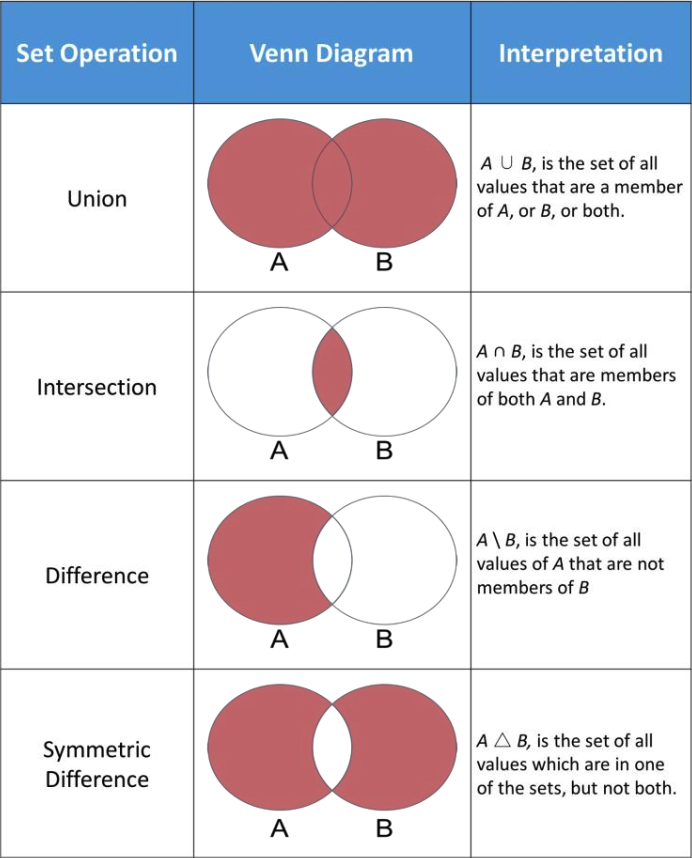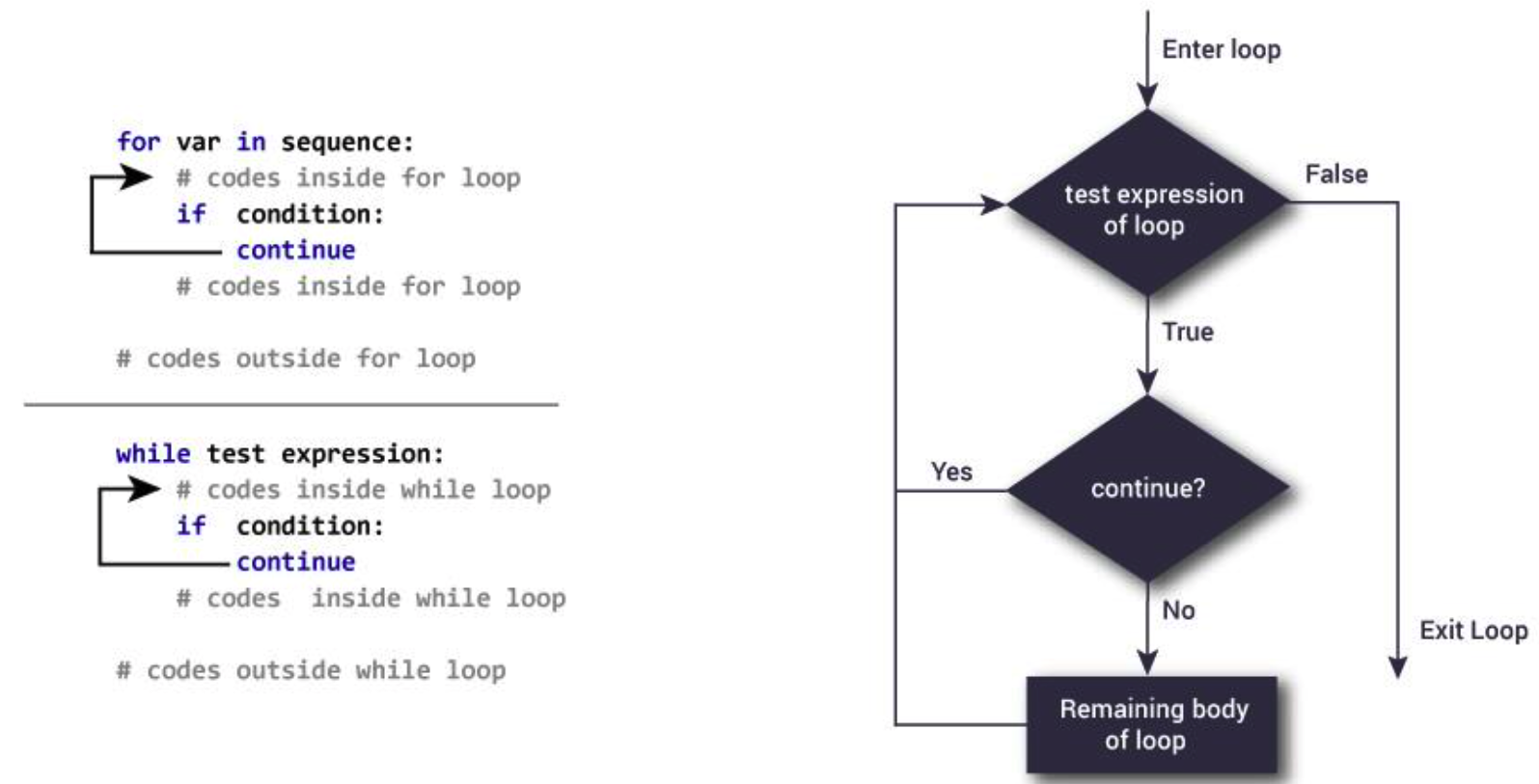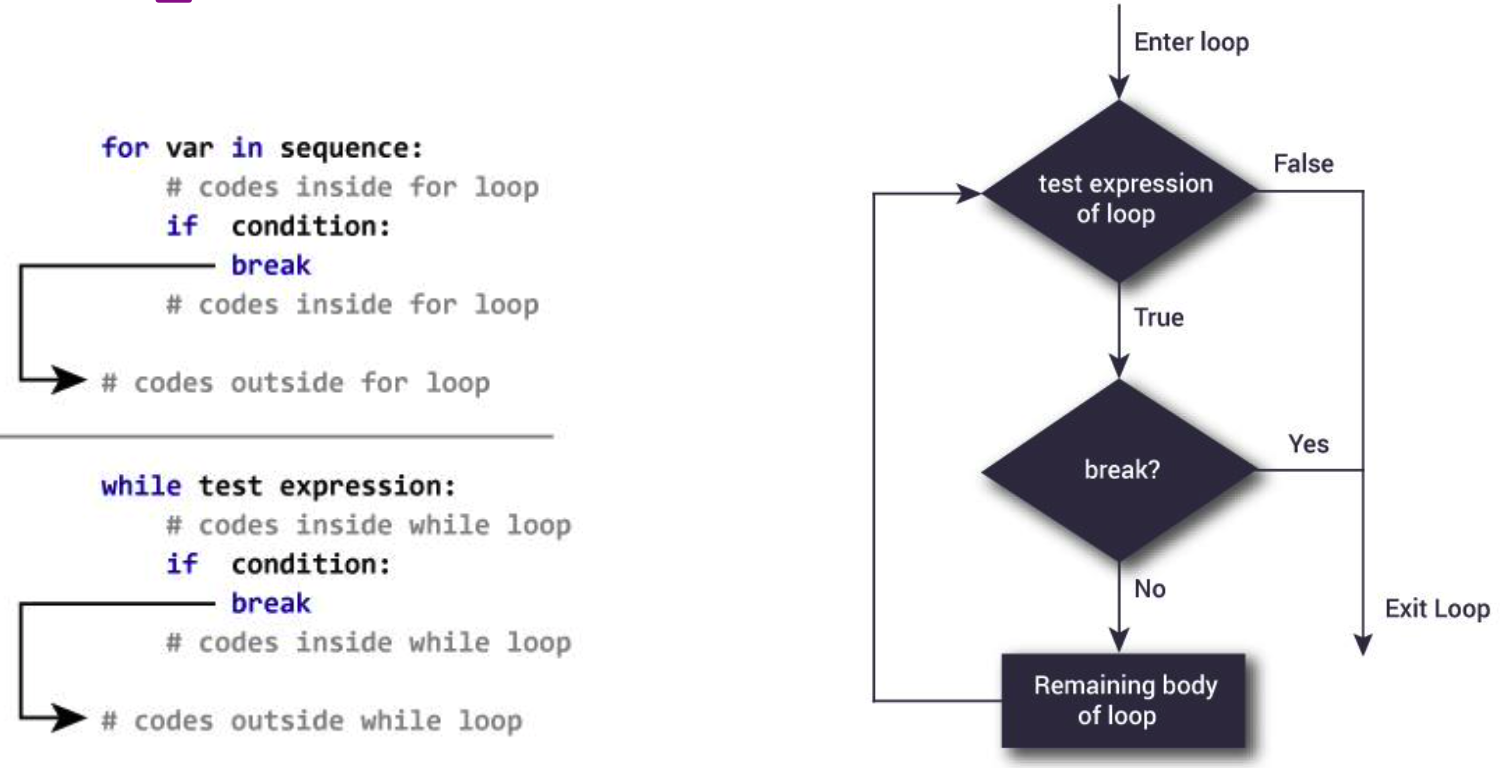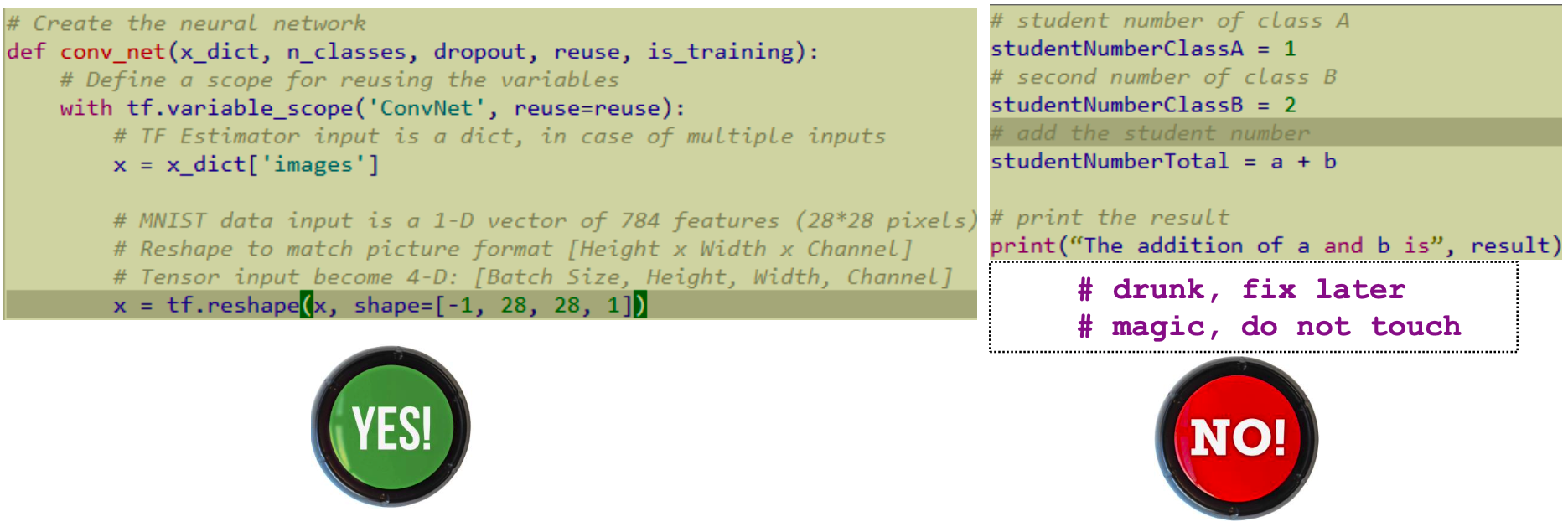ControlNet

## Python的集合数据类型

### 集合

• 空集合: a_set = set()
• 带有元素的集合: a_set = {"one", 2}
• 列表转换为集合: a_set = set([1,2,2,3]) 结果为 {1,2,3}

• a_set.add("c")

• a_set.remove(item) 接受一个参数，从这个集合中删除它，如果没有这个元素会抛出KeyError异常
• a_set.discard(item)a_set.remove一样删除元素，但是不会抛出异常
• a_set.pop() 随意返回一个元素并从集合中删除
• a_set.clear() 删除全部元素• 并集(Union)
• a_set.union(b_set)
• a_set | b_set
• 交集(Intersection)
• a_set.intersection(b_set)
• a_set & b_set
• 差集(Difference)
• a_set.difference(b_set)
• a_set - b_set
• 对称差(Symmetric difference)
• a_set.symmetric_difference(b_set)
• a_set ^ b_set

• 顺序
• 列表是有序的，集合是无序的
• 重复
• 列表允许重复元素，集合中没有重复元素
• in语句中
• 集合会比列表的速度快得多

### 字典

• 空字典: a_dict = {}
• 带有元素的字典: a_dict = {"one": 1, "two": 2}
• 从键值对元组列表中创建: a_dict = dict([("a", 1), ("b", 1)])

• a_dict[new_key] = new_value
• 如果new_key已经存在于这个字典中了，则会用new_value覆盖原先的value。

• del a_dict[a_key]

• a_key in a_dict 或者 a_key not in a_dict

## 循环控制

### Continue

Python中的continue关键字用于跳过循环中的这一轮，并且开始下一轮循环。### Break

Python中的break关键字将会直接跳出这个循环。## 注释

Python中的注释:

• #作为开头
• 注释的部分将不会被解释运行

• 在代码的同一行写上注释
• r = 1 # radius of the circle

• 一整行都是注释
• # some comment

• 多行注释，使用三引号"""
• 一般多用于代码的开头介绍，或者函数和类的介绍• 讨厌在自己的代码中写注释
• 讨厌别人的代码中没有注释

## 标准输入输出

### 标准输入

input用于从键盘接受输入的数据。

• input("prompt statement:") 或者 input()
• input是一个Python的内置函数，它的返回值是str。如果想要转换为int可以使用int()函数。

### 标准输出

print函数:

• print("output string")
• 默认情况下是用"\n"换行符结尾，所以每一个print语句会显示为一行。

• print中使用两个参数
• print("The addition of a and b is", result)
• 将两个参数重新拼接为一个字符串
• print("The addition of a and b is " + str(result))
• 注意，这里的+是用于拼接字符串，是Python内置的功能，加号两侧都需要是字符串类型。